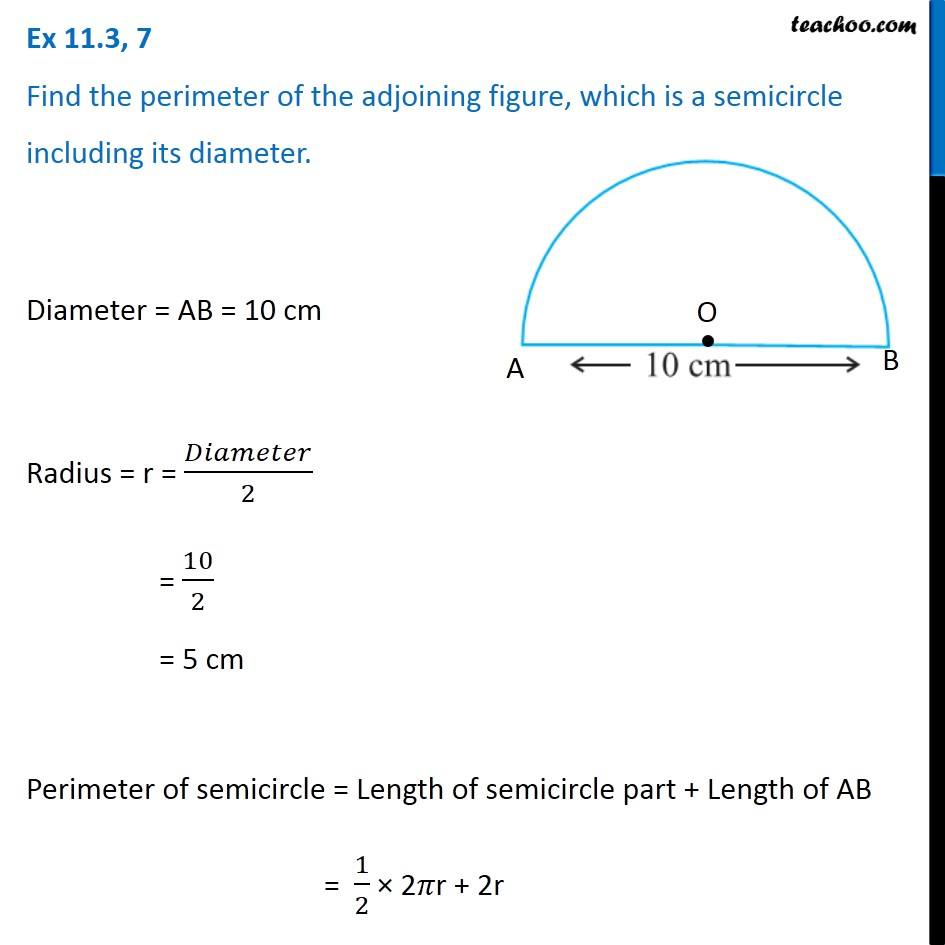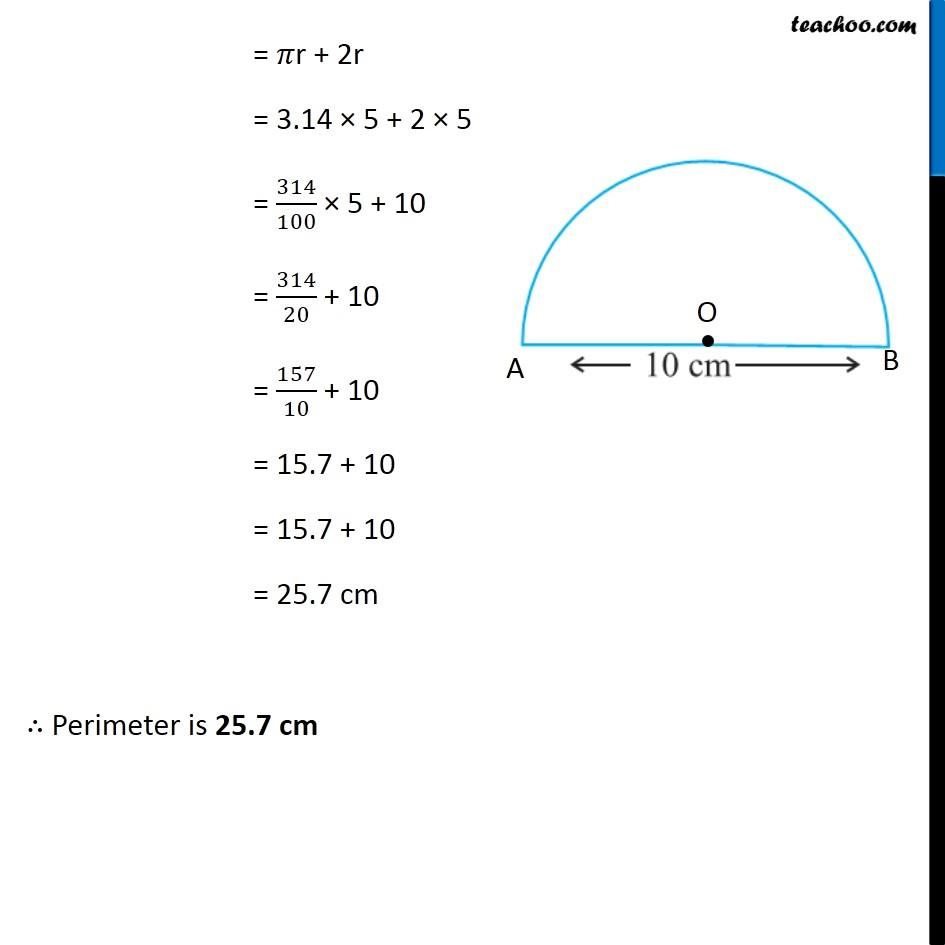1. Chapter 11 Class 7 Perimeter and Area
2. Concept wise
3. Perimeter of a combination of shapes

Transcript

Ex 11.3, 7 Find the perimeter of the adjoining figure, which is a semicircle including its diameter. Diameter = AB = 10 cm Radius = r = 𝐷𝑖𝑎𝑚𝑒𝑡𝑒𝑟/2 = 10/2 = 5 cm Perimeter of semicircle = Length of semicircle part + Length of AB = 1/2 × 2𝜋r + 2r = 𝜋r + 2r = 3.14 × 5 + 2 × 5 = 314/100 × 5 + 10 = 314/20 + 10 = 157/10 + 10 = 15.7 + 10 = 15.7 + 10 = 25.7 cm ∴ Perimeter is 25.7 cm

Perimeter of a combination of shapes